## GOING IN CIRCLES [DAY 3]

### EXPLORING PI: CIRCUMFERENCE & AREA OF CIRCLES

Leverage contextual problems to investigate and emerge Pi to help conceptualize formulas for both the circumference and are of a circle.

## Intentionality & Unit Overview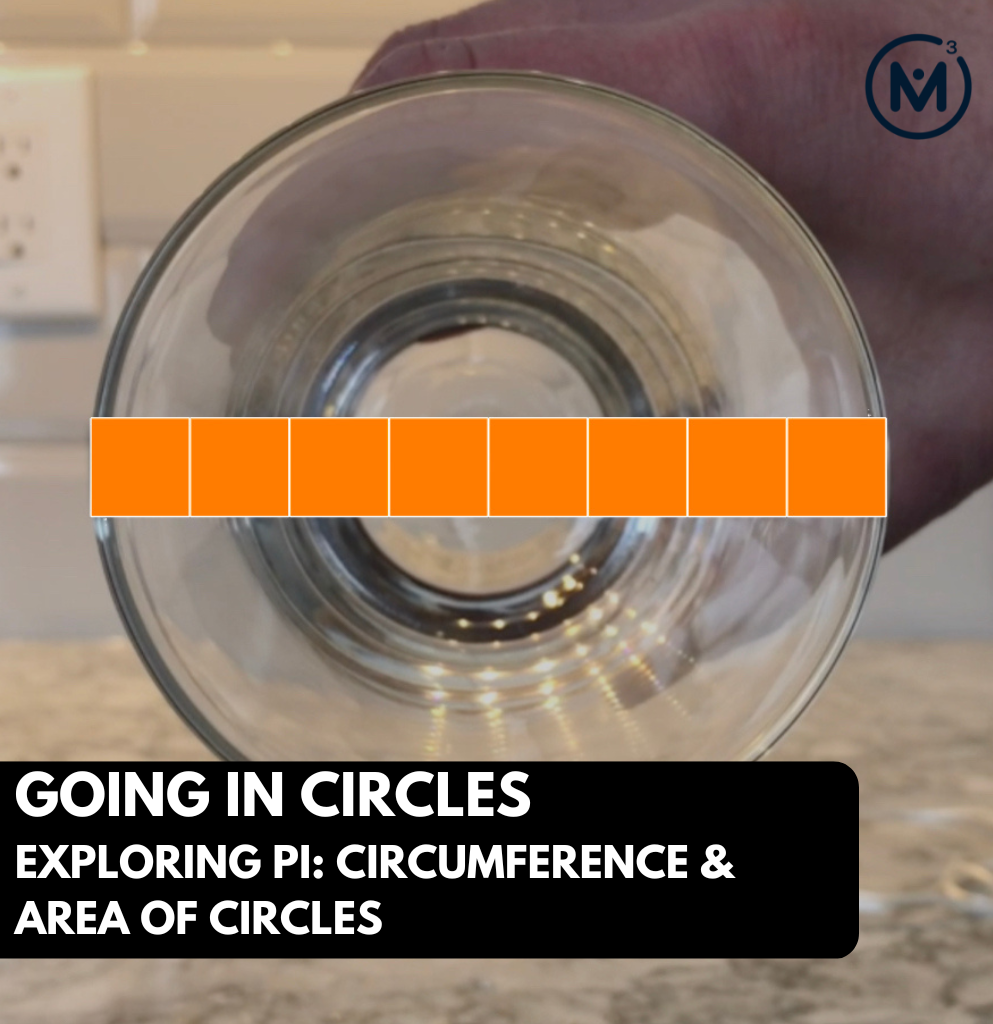### Access each lesson from this unit using the navigation links below

Students will apply the relationship between circumference and diameter of a circle known as Pi to find the unknown circumference given the diameter or radius of a circle or to find the unknown diameter or radius of a circle given the circumference.

Through this purposeful practice, students will apply the concept of circumference to deepen understanding and begin building procedural fluency related to big ideas including the following:

• When exploring measurement relationships of any circle, there are three (3) standard measures that can be used to reveal more information about the circle:
• Circumference: the perimeter of a circle.
• Diameter: any straight line segment beginning and ending on the outer edge of a circle and passes through its centre.
• Radius: any straight line segment beginning at the centre of a circle and ending on the outer edge of the circle.
• Knowing one of the standard measures of a circle provides information about all other measures;
• There is a multiplicative relationship between the length of the diameter and the circumference of a circle. In other words, as the diameter of a circle increases, the circumference increases multiplicatively (not additively);
• For all circles, the ratio of the circumference to the diameter is the same (approximately 22:7); and,
• For all circles, the rate of each unit of circumference length per unit of diameter length is the same (approximately $$\frac{22}{7}$$ or 3.14) and is known as Pi, π.

## Math Talk

In the number talk for today, students will be given an opportunity to build their multiplicative number flexibility by estimating the circumference of different circles beginning with a very small diameter that gradually increases from prompt to prompt.

Through this work with the approximate 3.14 to 1 ratio between the circumference and the diameter of any circle, students will be encouraged to explore the double number line model or ratio table and apply the use of partial products made possible with the distributive property through the following string of related problems.
It can be helpful to craft a context that students can leverage throughout this string. For example, using the Tile Circle context from Day 2 could be helpful where each problem is an expression beginning with the multiplicative comparison between the circumference and the diameter (Pi, π) and the diameter in square tiles.

You might consider facilitating this number talk orally or consider leveraging the visual number talk prompts included to assist in your facilitation. Here is the string of number talk prompts:

What about how long is the circumference of a circle with a diameter of…

2 units?

4 units?

8 units?

24 units?

48 units?

Regardless of whether you choose to orally facilitate this number talk or leverage the visual number talk prompts, be sure to record student thinking publicly for all to see including the symbolic representation of the work they are engaging in from this string as you go.

3.14 x 2

3.14 x 4

3.14 x 8

3.14 x 24

3.14 x 48

Encourage or create opportunities to represent student thinking using the double number line by pausing questions such as “would it be ok if I show your thinking using this double number line” if students do not initiate the use of a model. If students want to model their thinking another way however, this should be honoured not discouraged. Through this string, encourage students to recognize the multiplicative relationship between the circumference and diameter of a circle to make reasonable estimates when given the value of one measure with another unknown.

Also, it is worth highlighting that despite the fact that we have a multiplicative comparison ratio between the circumference and the diameter of a circle, we can also think of this relationship as a composed unit ratio since it feels natural to scale this ratio in tandem on the double number line or in the ratio table to emerge the circumference and/or diameter of other circles.

## Visual Math Talk Prompt #1

Begin playing the following visual number talk prompt video and be ready to pause the video to allow for think time.

Pause the video where indicated, and share the following prompt:

How many units will it take to wrap the circle?
Make an estimate.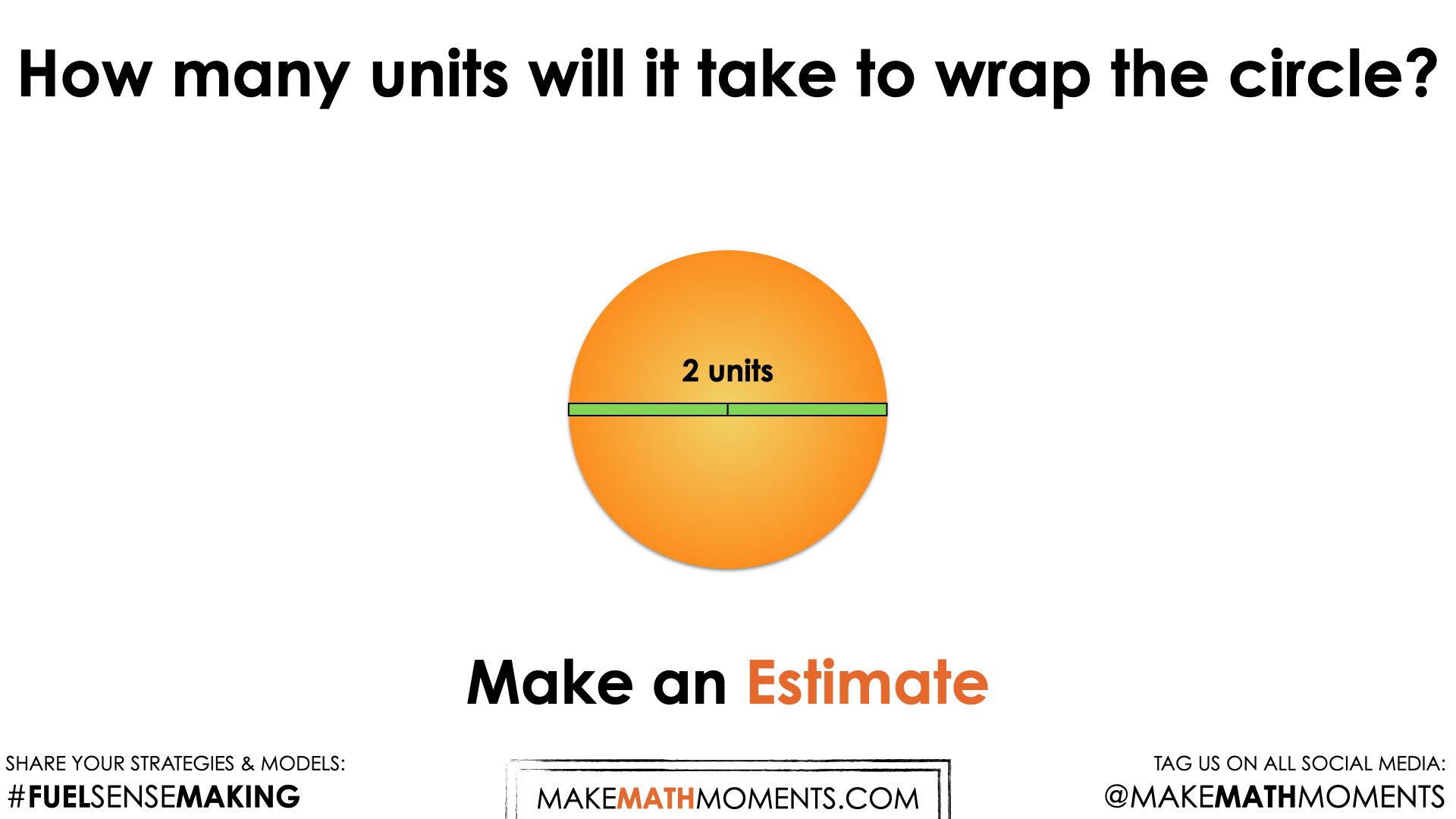Of course, be sure to let students know that you want them to be estimating without the use of a calculator and you want them to craft a convincing argument for how they came up with their estimate. This might include the use of mathematical models such as visual models, symbolic models or even share their thinking orally.

For some students who have participated in the first two days of this unit, they might recall that we learned the circumference of any circle is about 3 times longer than the diameter; a multiple known as Pi, π. Therefore, some students might be able to quickly estimate an answer of “about 6 units” for the circumference, while others might be able to come up with a more precise estimate given that they know they need approximately an additional 14 hundredths of the diameter. In this case, an additional 14 hundredths of each of the 2 units used to measure the diameter.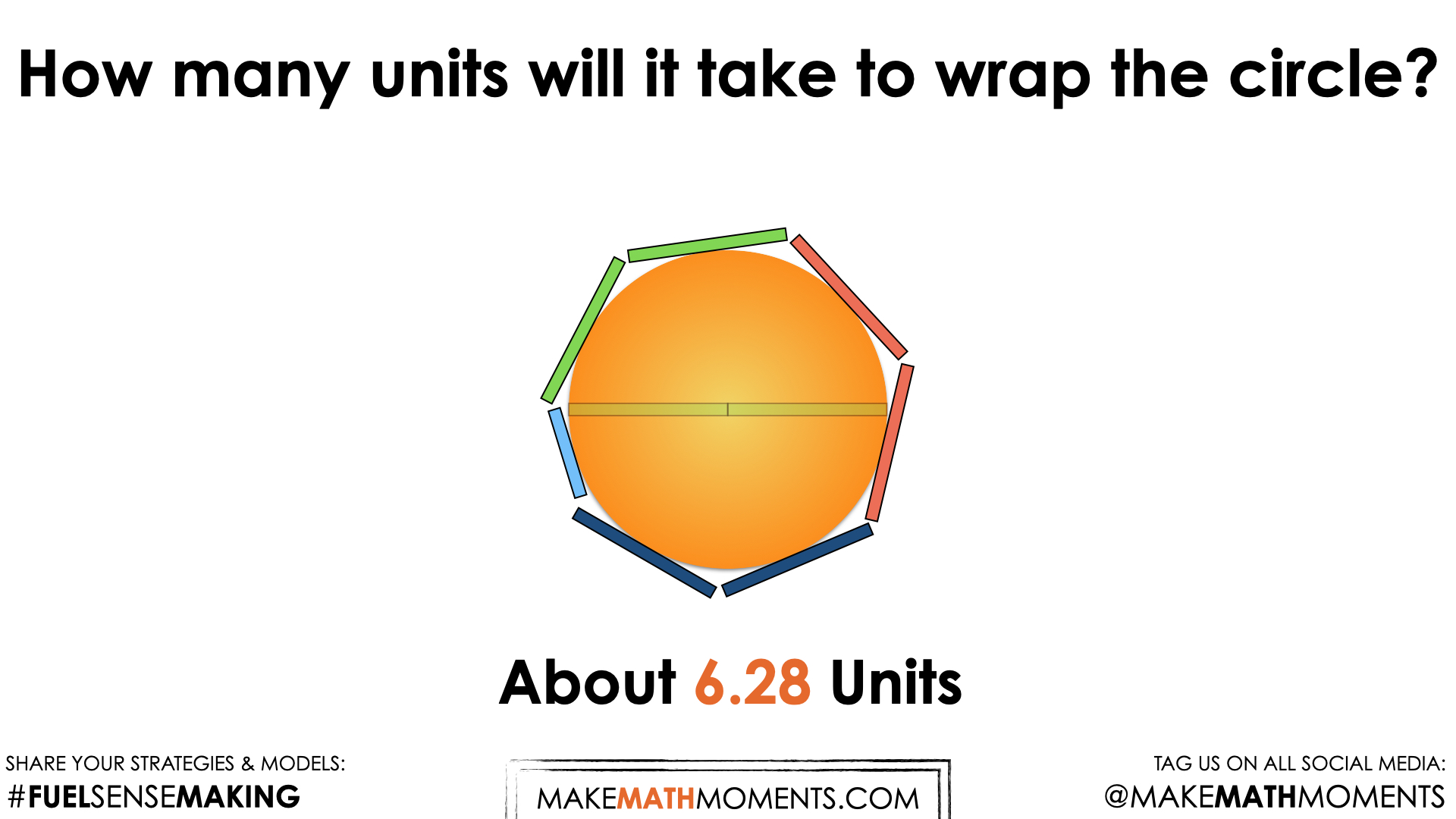It can be helpful to highlight that since a circle with a diameter of 1 unit would have a circumference of approximately 3.14 units, doubling our diameter would also double our circumference.

It can be helpful to highlight this on a double number line and/or in the ratio table.

Finally, highlighting that we can write this algebraically as C = πd and multiplying the diameter by a rate of 3.14 circumference units per diameter unit can also be beneficial.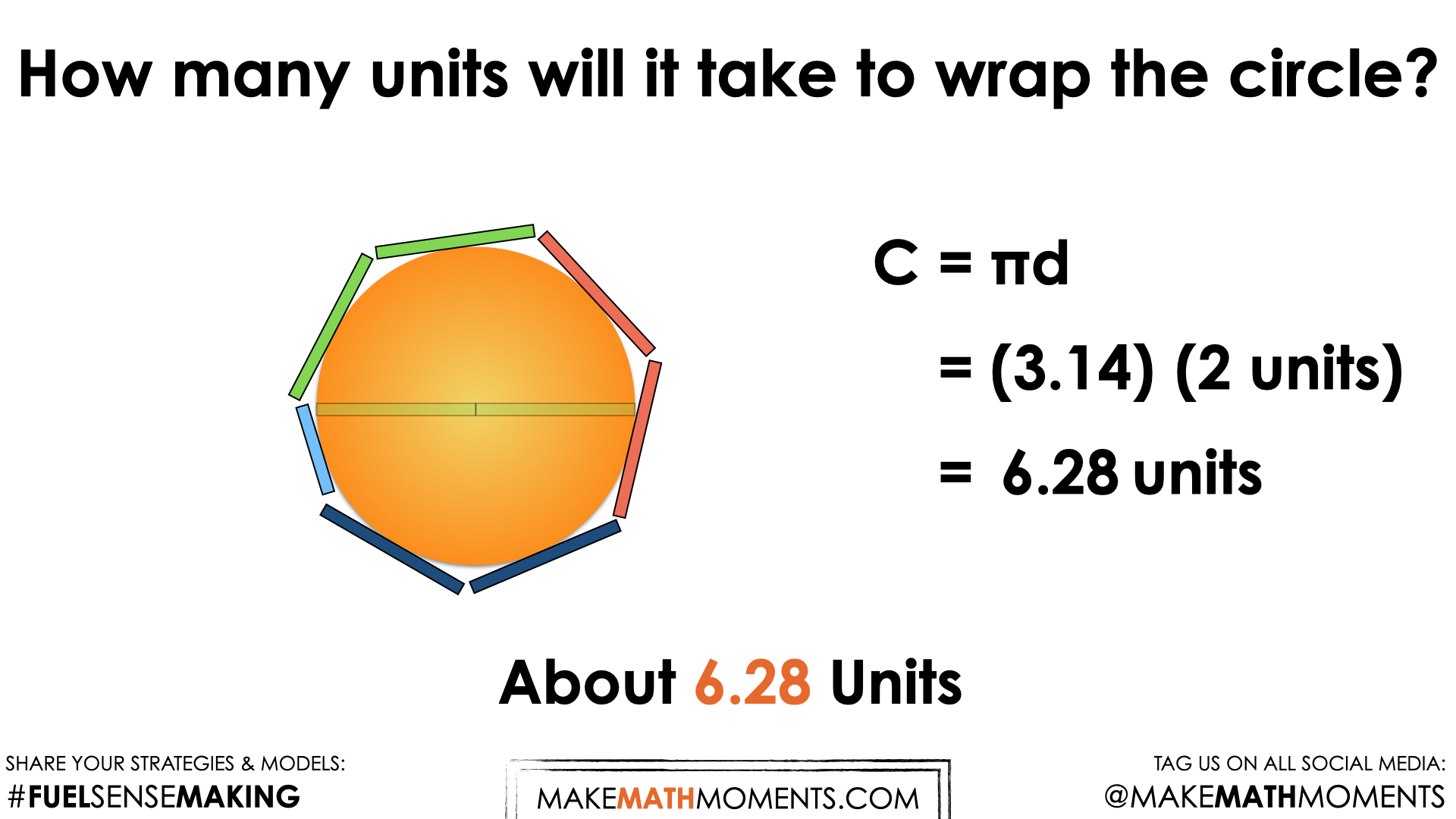## Visual Math Talk Prompt #2

Play the second visual number talk prompt video and be ready to pause the video to allow for think time.

Once again, pausing the video when the following prompt appears:

How many units will it take to wrap the circle?
Make an estimate.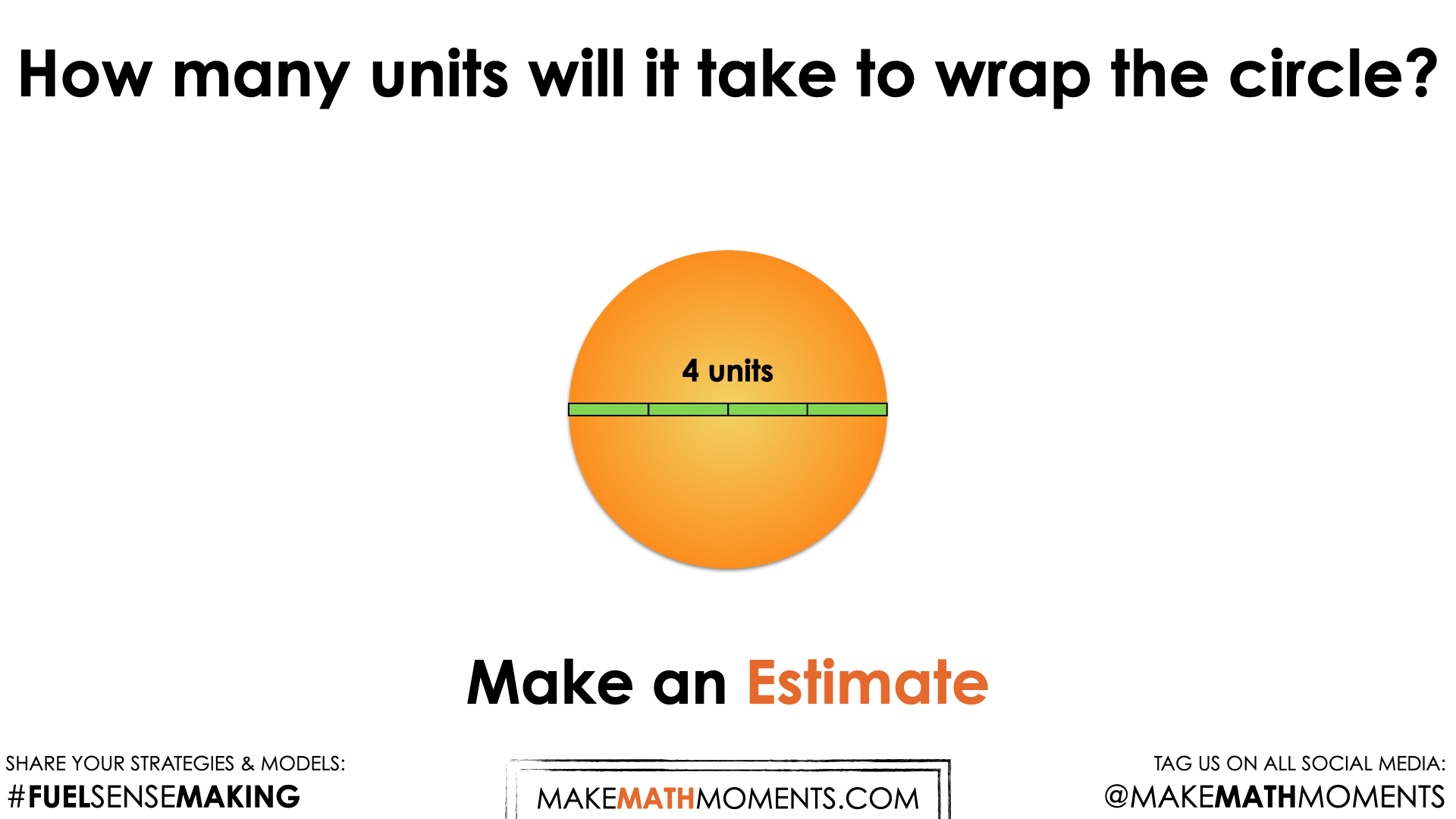As students begin making their estimates, be sure to monitor which students are actually leveraging their understanding of Pi, π, which students are leveraging the π to 1 composed unit ratio relationship to scale in tandem (i.e.: doubling the diameter and doubling the circumference from prompt #1) and which students are leveraging rate reasoning to multiply Pi, π as a rate by the diameter.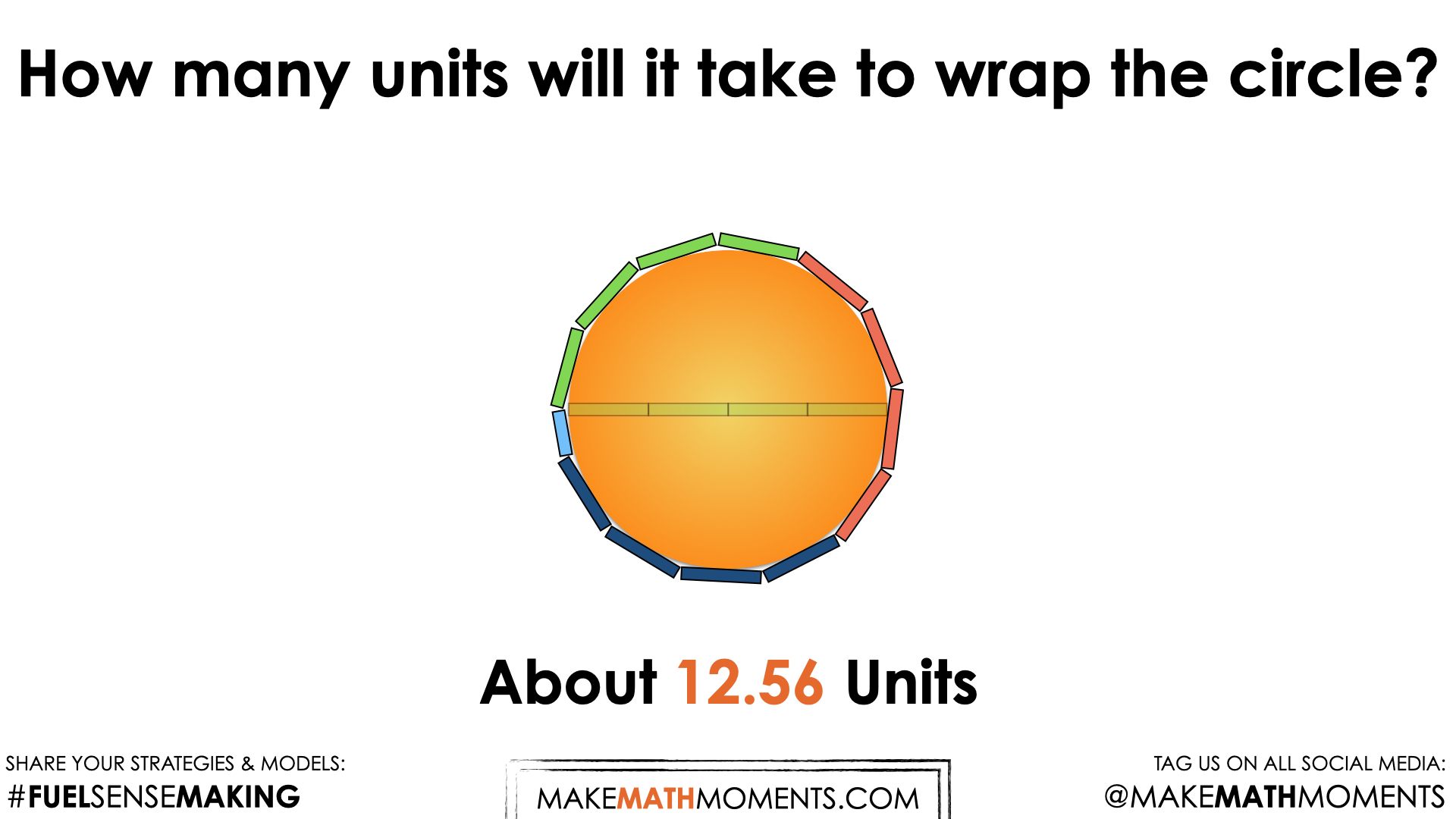Regardless of the method students choose, the goal is that all students share an estimate of between 12 and 13 units.

After modelling student ratio reasoning via scaling in tandem on a double number line and/or ratio table, ensure that you also highlight rate reasoning via leveraging the circumference formula as well.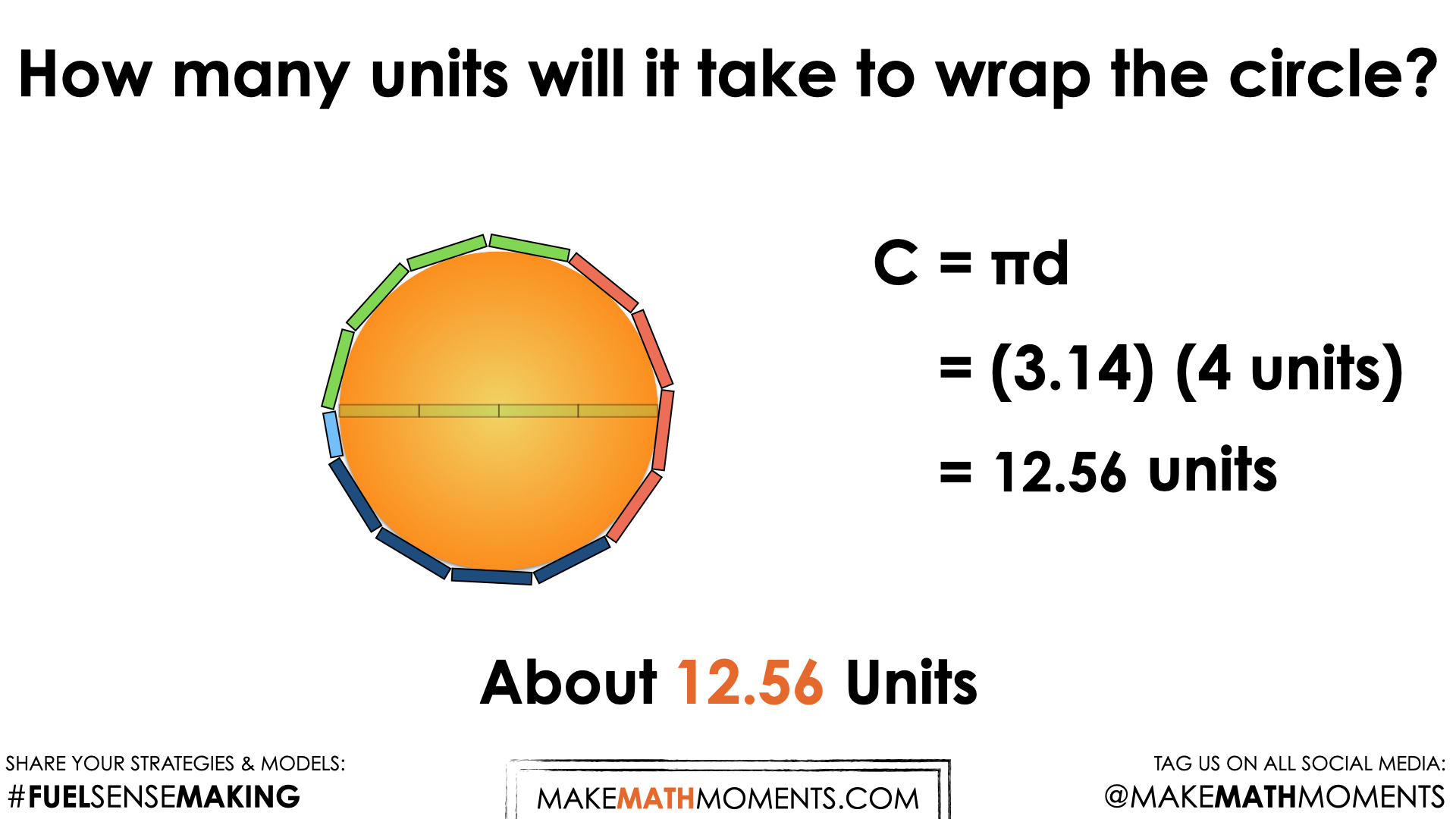## Visual Math Talk Prompt #3

Play the next visual number talk prompt video and be ready to pause the video to allow for think time.

Pause the video when the following prompt appears:

How many units will it take to wrap the circle?
Make an estimate.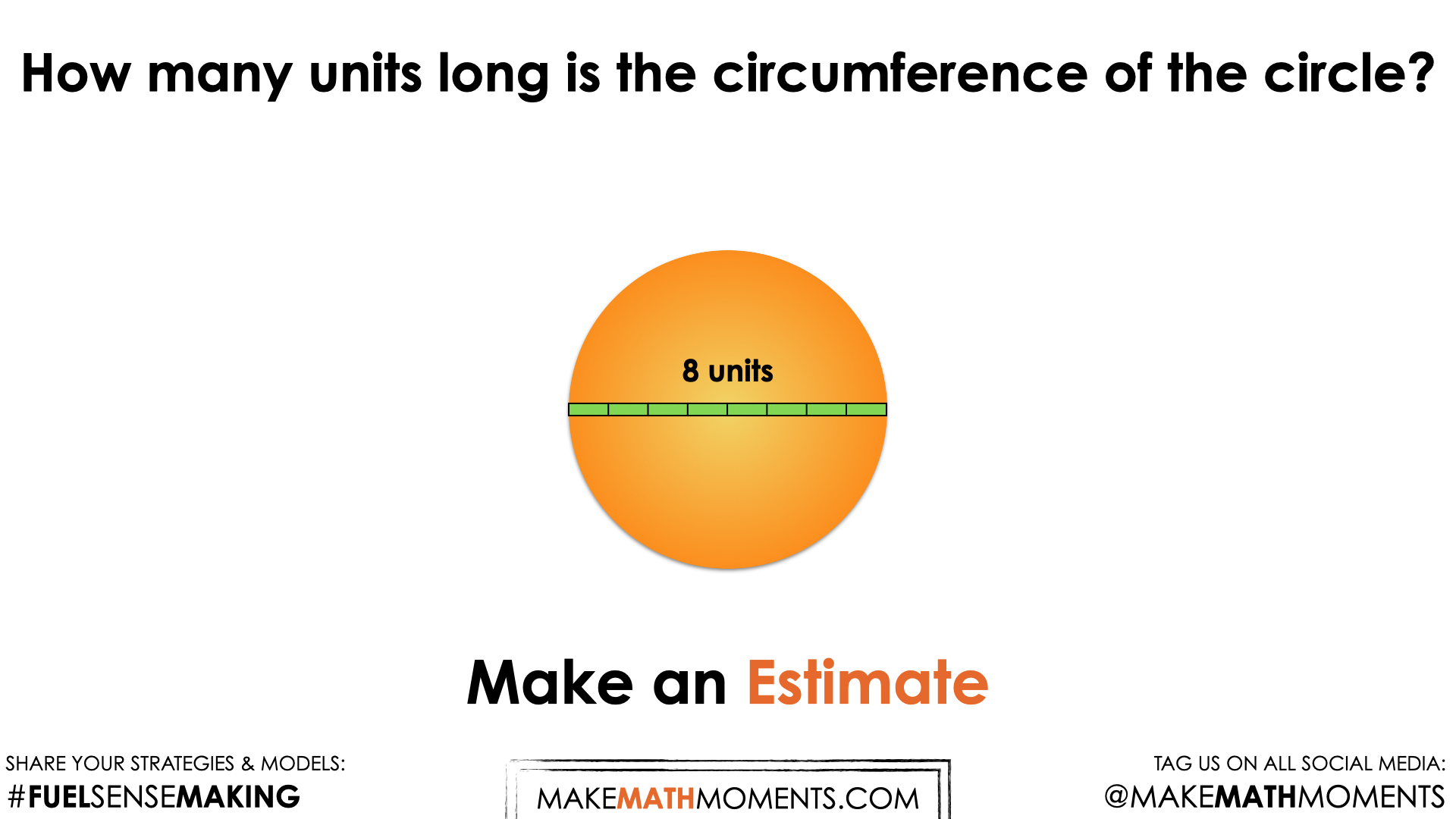Continue monitoring what strategy and mathematical model each student is leveraging as they work through this string. Can you encourage them to also show it using another approach?

If some students are consistently using a more friendly 3 instead of 3.14 as an approximation for Pi, π, consider modelling the multiplication of 3.14 by 8 units using partial products on the number line and/or with an area model.

For example, decomposing 3.14 into (3 + 0.14) allows for multiplying 3.14 by 8 units in two parts:

3.14 x 8 units
= (3 + 0.14) x 8 units
= (3 x 8 units) + (0.14 x 8 units)

This may or may not be more helpful for some students as multiplying 0.14 by 8 units might still be a challenge. However, we might leverage a doubling and halving strategy to help us make this calculation more friendly without the use of a calculator:

= (3 x 8 units) + (0.14 x 8 units)
= 24 units + (0.28 x 4 units)
= 24 units + (0.56 x 2 units)
= 24 units + (1.12 units)
= 25.12 units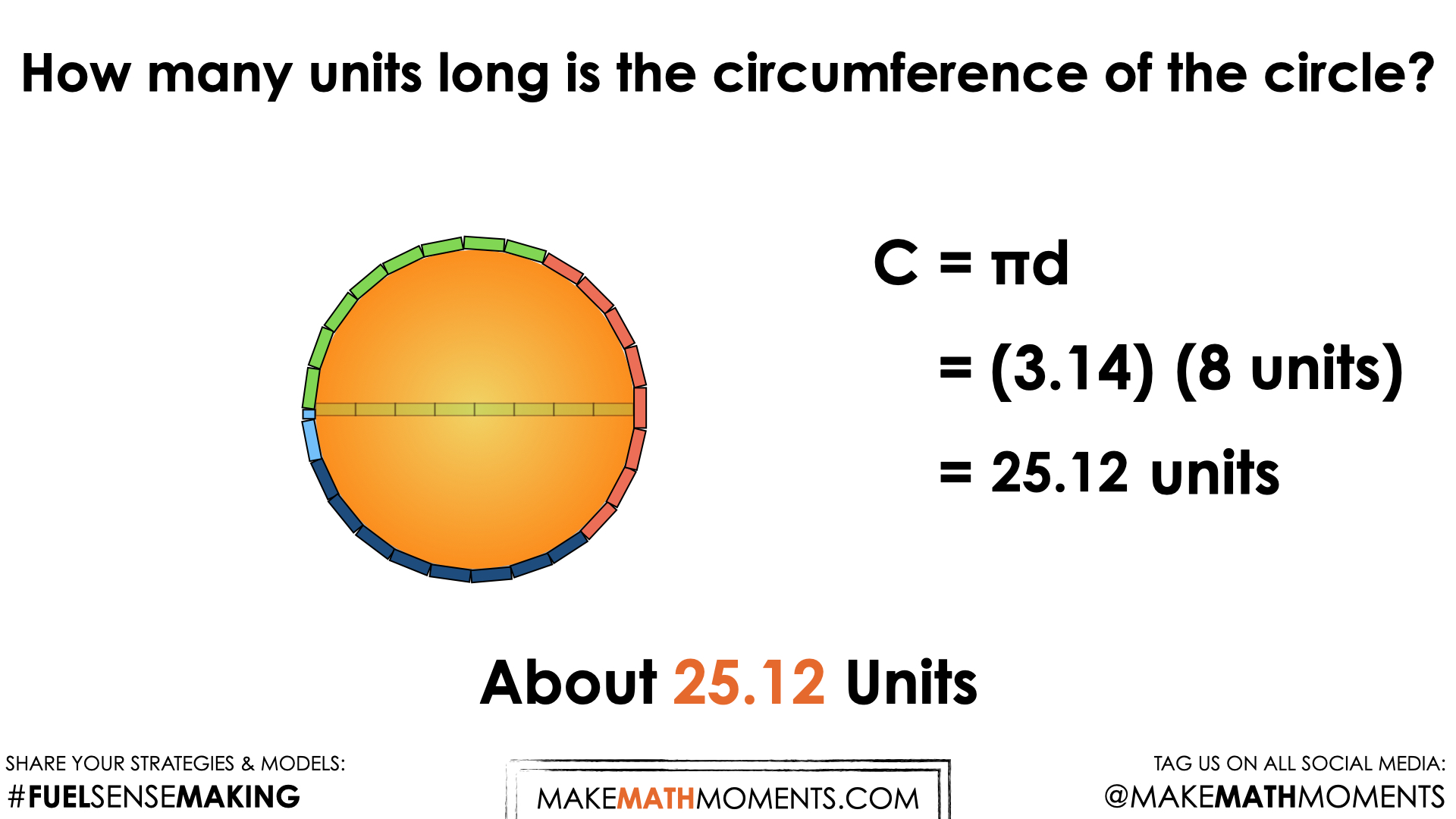## Visual Math Talk Prompt #4

Continue by playing the next visual number talk prompt video and be ready to pause the video to allow for think time.

Pause the video when the following prompt appears:

How many units will it take to wrap the circle?
Make an estimate.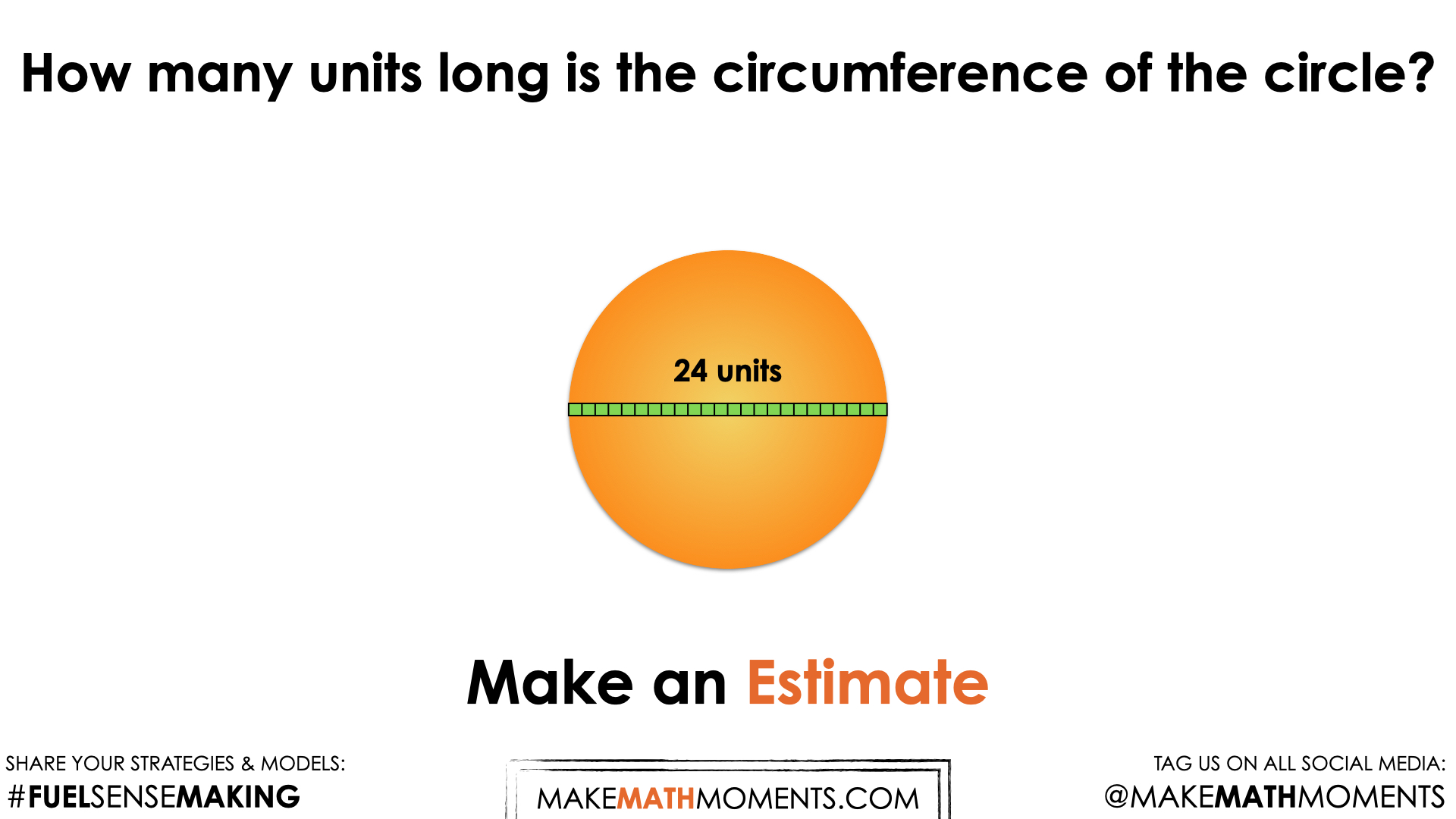For students who have been leveraging scaling in tandem, they might notice that our diameter has tripled from the previous prompt and thus the circumference will also triple.

Continue to document student approaches and do your best to represent their thinking leveraging mathematical models for other students to benefit from.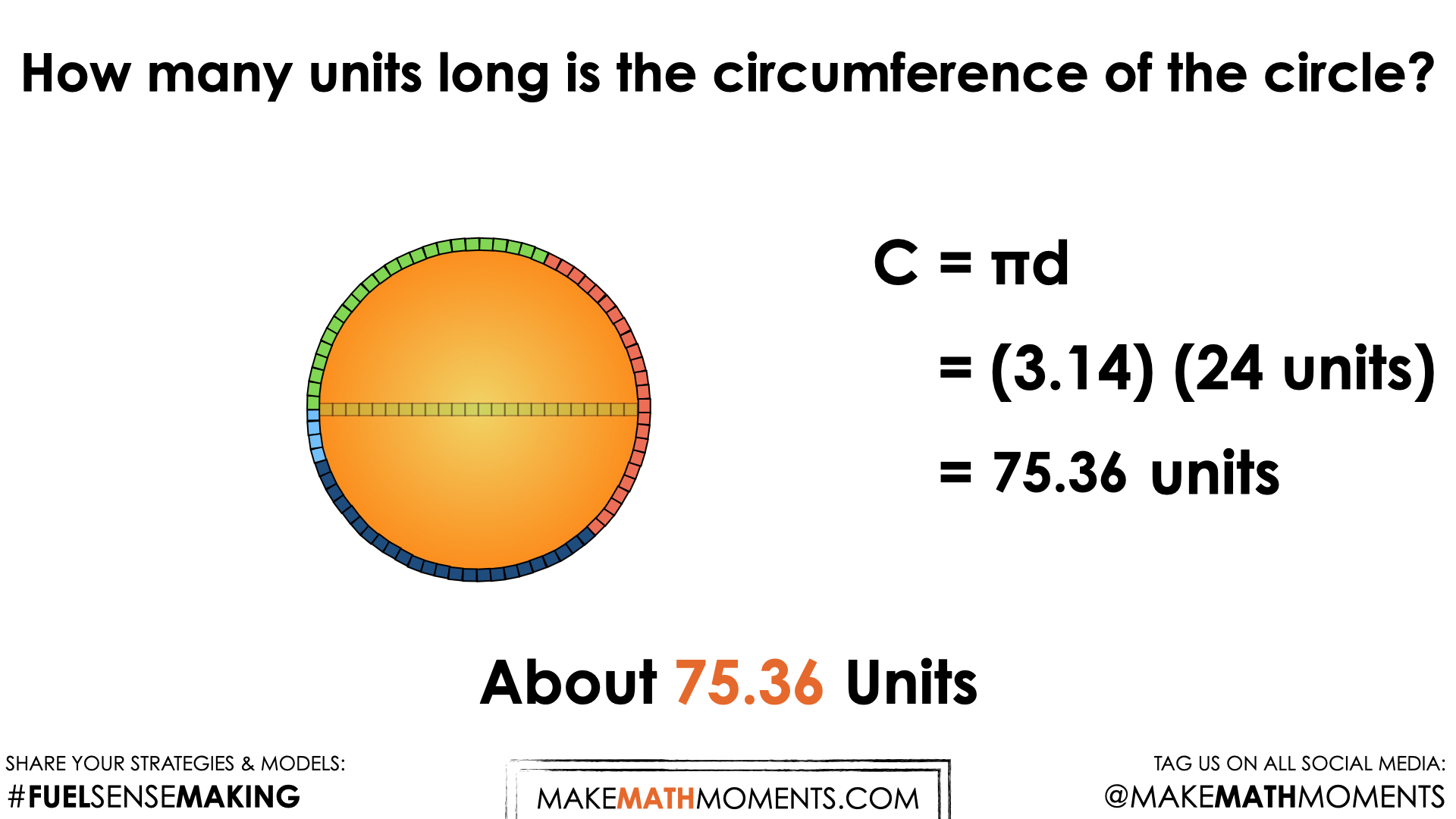## Visual Math Talk Prompt #5

Play the next visual number talk prompt video and be ready to pause the video to allow for think time.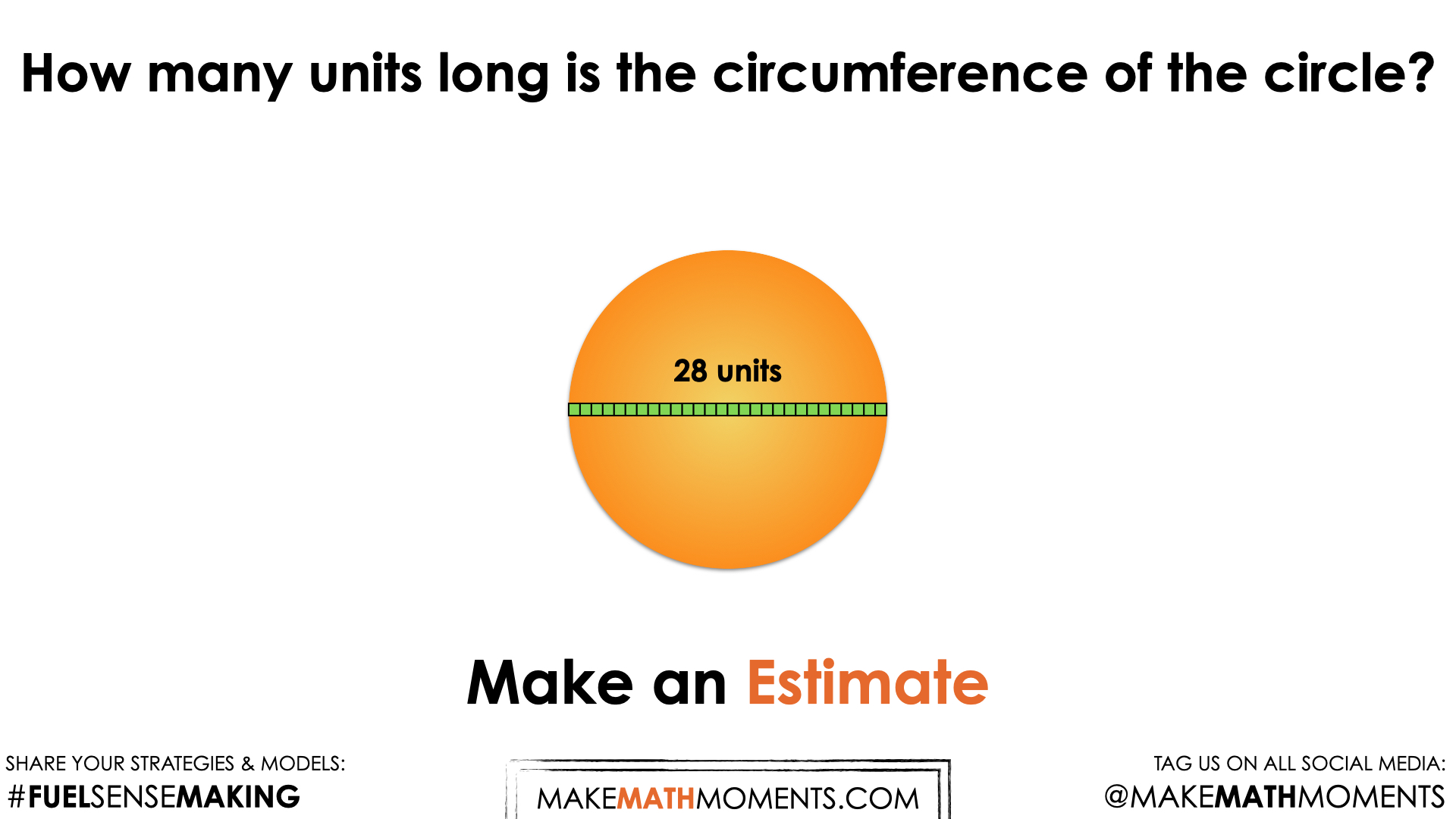In this prompt, students may quickly realize that our diameter increased by an unfriendly factor of the previous prompt. Something students may not realize is that the diameter is still increasing by a multiple of the previous diameter, however the multiple is not nearly as friendly (i.e.: a 28/24 or 14/12 or 7/6 multiple). This might be worth highlighting if students falsely believe that the multiplicative relationship is no longer present.

Because scaling in tandem without a calculator is not friendly in this case, you might find more students resorting to rate reasoning by simply leveraging Pi, π as a rate to multiply by the diameter.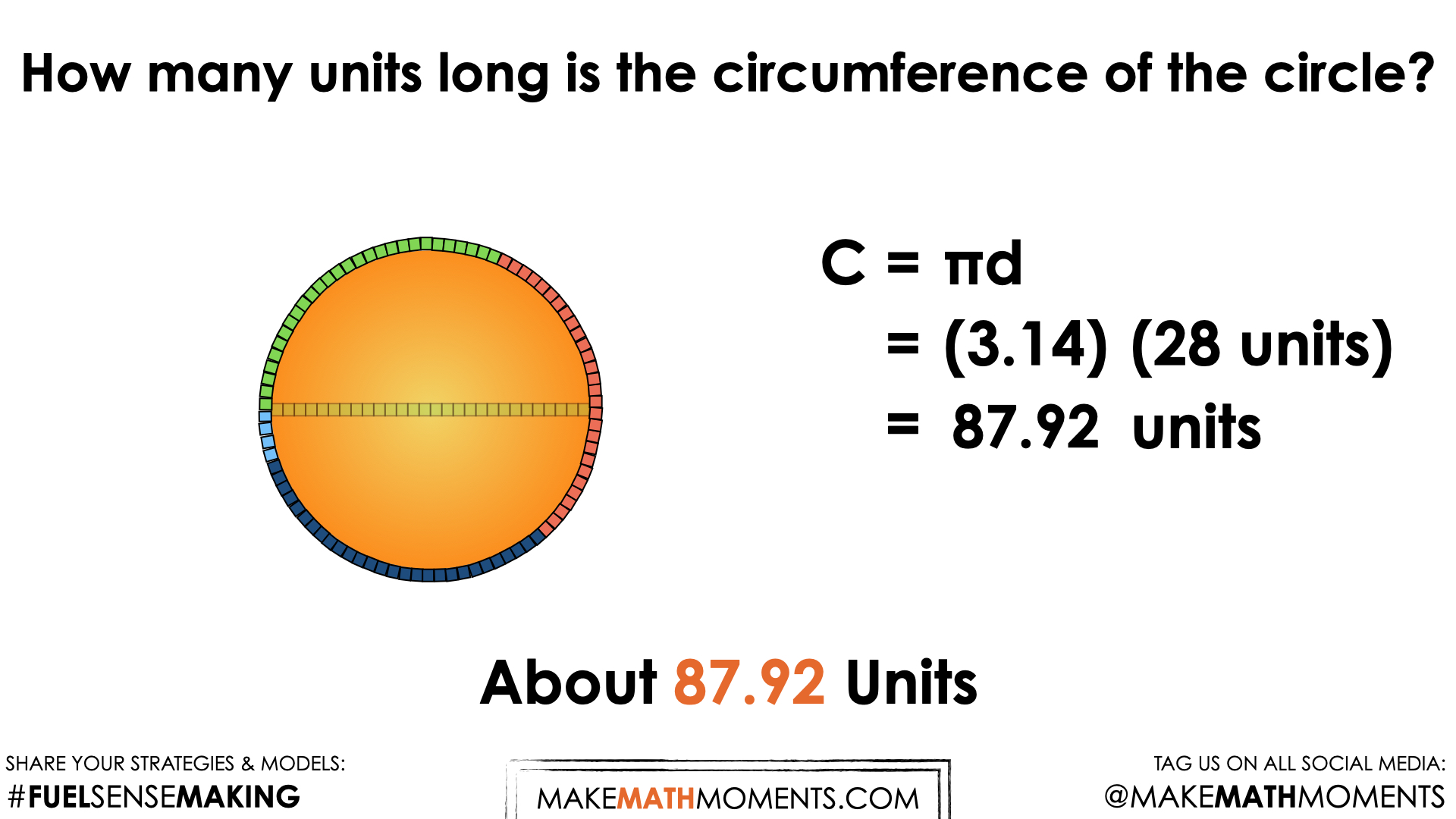## Visual Math Talk Prompt #6

Login/Join to access the full teacher guides, downloadable slide decks and printable handouts for this lesson and all problem based units.

## Visual Math Talk Prompt #7

Login/Join to access the full teacher guides, downloadable slide decks and printable handouts for this lesson and all problem based units.

## Working With Circles: Circumference

Login/Join to access the full teacher guides, downloadable slide decks and printable handouts for this lesson and all problem based units.

## Practice Problems: Circumference of a Circle

Login/Join to access the full teacher guides, downloadable slide decks and printable handouts for this lesson and all problem based units.

Login/Join to access the entire Teacher Guide, downloadable slide decks and printable handouts for this lesson and all problem based units.

## Educator Discussion Area

Login/Join to access the entire Teacher Guide, downloadable slide decks and printable handouts for this lesson and all problem based units.

## Explore Our 60+ Problem Based Units

This Make Math Moments Lesson was designed to spark curiosity for a multi-day unit of study with built in purposeful practice, number talks and extensions to elicit and emerge strategies and mathematical models.

Dig into our other units of study and view by concept continuum, grade or topic!